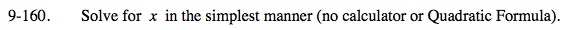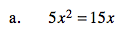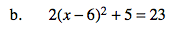### Home > PC > Chapter 9 > Lesson 9.3.5 > Problem9-160

9-160.
1.Solve for x in the simplest manner (no calculator or Quadratic Formula). Homework Help ✎

1. 5x2 = 15x

2. 2(x − 6)2 + 5 = 231. Do NOT divide both sides by x. This will eliminate one of your two answers.
2. Instead, move all terms to one side and set = to 0
Factor. Solve.2(x − 6)2 = 18
(x − 6)2 = 9
x − 6 = ± 3
x = ±3 + 6
x = 9 x = 3